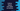# Python degree and radian conversion# Degree and radian in python :

math module in python provides two different methods for a degree to radian and radian to degree conversion. Degree and radian are used for angle measurement and conversion of one to another are frequently used in applications like weather apps. You can write your own function to do the conversion but the math module already provides these functions. In this tutorial, I will show you how to use these math module functions.

## degrees() :

math.degrees() takes the radians value and returns its degree equivalent. It takes the radians value as the parameter.

``````import math

print("Radians of {} is : {}".format(90, math.degrees(90)))
print("Radians of {} is : {}".format(0, math.degrees(0)))
print("Radians of {} is : {}".format(180, math.degrees(180)))``````

It will print the below output :

``````Radians of 90 is : 5156.620156177409
Radians of 0 is : 0.0
Radians of 180 is : 10313.240312354817``````

This method takes the value of the degree as its parameter and returns the radians equivalent. Similar to degrees, it also returns a float value. For example :

``````import math

print("Degrees of {} is : {}".format(
``````Radians of 5156.620156177409 is : 90.0